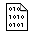## Firefly and PC GAMESS-related discussion club

Learn how to ask questions correctlyRe^3: Problems with SA-MCSCF Geometry optimization
Hi,
I am attaching a second example where I have found
a similar behaviour. The Energy seems to go down and then increases.

On Thu May 16 '13 8:56am, lello wrote
-------------------------------------
>Hi,
>I have attached the input.
>
>
>On Wed May 15 '13 11:31pm, Alex Granovsky wrote
>-----------------------------------------------
>>Hello,

>>Kind regards,
>>Alex Granovsky
>>
>>
>>On Wed May 15 '13 10:55pm, lello wrote
>>--------------------------------------
>>>Dear all,

>>>I am trying to perform a SA-MCSCF geometry optimization
>>>of the ground state of a system, and I have the following behaviour
>>>of the energy of the target state:

>>> NSERCH=  0     ENERGY= -915.9734212   RMS GRAD = 0.0095239
>>>  NSERCH=  1     ENERGY= -915.9501045   RMS GRAD = 0.0196205
>>>  NSERCH=  2     ENERGY= -915.9576756   RMS GRAD = 0.0058206
>>>  NSERCH=  3     ENERGY= -915.9634734   RMS GRAD = 0.0091716
>>>  NSERCH=  4     ENERGY= -915.9626773   RMS GRAD = 0.0056877
>>>  NSERCH=  5     ENERGY= -915.9597202   RMS GRAD = 0.0036023
>>>  NSERCH=  6     ENERGY= -915.9586388   RMS GRAD = 0.0022706
>>>  NSERCH=  7     ENERGY= -915.9581777   RMS GRAD = 0.0020863
>>>  NSERCH=  8     ENERGY= -915.9575078   RMS GRAD = 0.0033306
>>>  NSERCH=  9     ENERGY= -915.9566032   RMS GRAD = 0.0036036
>>>  NSERCH= 10     ENERGY= -915.9559209   RMS GRAD = 0.0035010
>>>...
>>>-----
>>>As you can see the first point is quite low in energy but after that
>>>the energy increase a lot. I have let the optimization run for more than 100 cycles but the energy is always high and never reaches
>>>that of the first point.
>>>I have observed this behaviour in a several
>>>cases. Is it just a problem of coordinate choice for the optimization
>>>or am I doing something wrong?
>>>Below is my input:

>>> \$CONTRL SCFTYP=MCSCF RUNTYP=OPTIMIZE MAXIT=120
>>>         D5=.T. FSTINT=.T. GENCON=.T. ICHARG=1 MULT=2 INTTYP=HONDO
>>>         ICUT=11   ITOL=20 \$END
>>> \$SCF DIRSCF=.TRUE. \$END
>>> \$SYSTEM TIMLIM=1000000 MEMORY=100000000 KDIAG=0 NOJAC=100 \$END
>>> \$P2P P2P=.T. DLB=.T. \$END
>>> \$TRANS MPTRAN=2 DIRTRF=.T. AOINTS=DIST ALTPAR=.T. MODE=112 \$END
>>> \$BASIS EXTFIL=.T. GBASIS=cc-pVDZ \$END
>>> \$MCSCF CISTEP=ALDET SOSCF=.T.  NUMFO=6 MAXIT=100 ACURCY=1.0E-7 \$END
>>> \$MCSCF NTRACK=2 ISTATE=1 \$END
>>> \$MCSCF NPFLG(9)=1 \$END
>>> \$DET NCORE=66 NACT=4 NELS=3 NSTATE=4 ITERMX=400
>>>     WSTATE(1)=1.0,1.0 CVGTOL=1.0E-7 \$END
>>> \$PCM SOLVNT=METHYCL \$END
>>> \$STATPT NSTEP=200 METHOD=GDIIS OPTTOL=0.0015 NPRT=1 NPUN=2 \$END
>>>
>>>This message contains the 2951 kb attachment [ cas-pcm.tar.gz ]Thu May 16 '13 11:02amThis message read 818 times